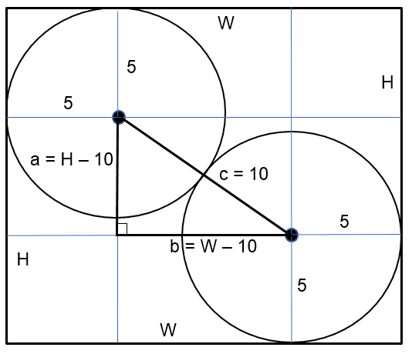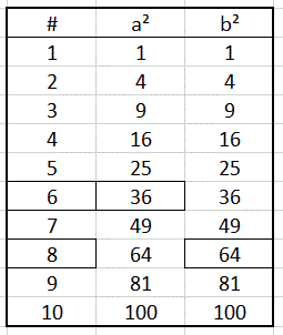Select Page

Each month, a new set of puzzles will be posted.  Come back next month for the solutions and a new set of puzzles, or subscribe to have them sent directly to you.

## EQUATE+3 Puzzle

Each row, column and diagonal is an equation and you use the numbers 1 to 9 to complete the equations. Each number can be used only once. Three numbers have been provided to get you started. Find the remaining six numbers that satisfies all the resulting equations. Note: multiplication (x) and division (/) are performed before addition (+) and subtraction (-)## EQUATE+1 Puzzle

Each row, column and diagonal is an equation and you use the numbers 1 to 9 to complete the equations. Each number can be used only once. One number has been provided to get you started. Find the remaining eight numbers that satisfies all the resulting equations. Note: Multiplication (x) and division (/) are performed before addition (+) and subtraction (-).## Feedback

There are more than one way of doing these puzzles and may well be more than one answer.  Please let me and others know what alternatives you find by commenting below.  We also welcome general comments on the subject and any feedback you'd like to give.

If you have a question that needs a response from me or you would like to contact me privately, please use the contact form.

## Get more puzzles!

If you've enjoyed doing the puzzles, consider ordering the books;

• Book One - 150+ of the best puzzles
• Book Two - 200+ with new originals and more of your favourites

Both in a handy pocket sized format.   Click here for full details.

## MIND-Xpander Challenges

Note: The short cut formula for ‘Adding Consecutive Numbers in a Sequence’ is as follows:

1. Add the smallest number in the consecutive group to the largest
2. Multiply the result by the total numbers in the group and finally, divide the product by 2.

Q1. What would the total be if you add ‘all’ the numbers in the first 200 consecutive numbers (i.e., 1 + 2 + 3 + 4 + 5 + etc.)?

Answer: [(1 + 200) x 200] / 2 = 40200 /2 = 20,100

Q2. What would the total be if you add all the ‘even’ numbers in the first 200 consecutive numbers (i.e., 2 + 4 + 6 + 8 + 10 + etc.)?

Answer: [(2 + 200) x 100] / 2 = 20200 /2 = 10,100

Q3. What would the total be if you add all the ‘odd’ numbers in the first 200 consecutive numbers (i.e., 1 + 3 + 5 + 7 + 9 + etc.)?

Answer: [(1 + 199) x 100] / 2 = 2000 /2 = 10,000

## MIND-Xpander  (‘Circles in a Rectangle’ Challenge – Level 1)

The diagram below shows two identical circles touching each other and completely put inside a rectangle (as shown below). The circles are not over-lapping and each touches two sides of the rectangle (as shown). What are the of dimensions of the rectangle if their radius is 5cm? Note: The diagram is not drawn to scale.

Solution:

Using the Pythagorean Equation, a² + b² = c², so a² + b² = (10)², or a² + b² = 100.Using the table of values, a² (36) + b² (64) = c² (100) satisfies the equation.Therefore:

a = 6, b = 8 & c = 10 and
H = a + 10 = 6 + 10 = 16 and
W = b + 10 = 8 + 10 = 18